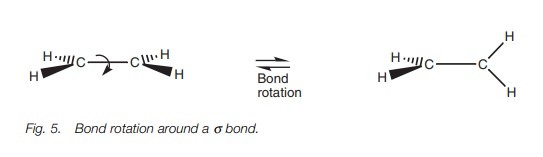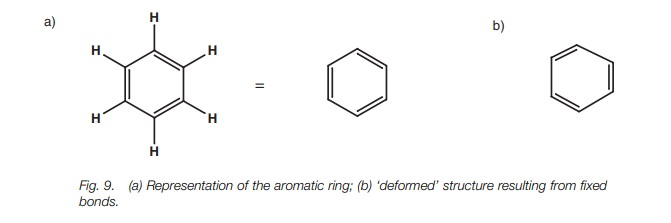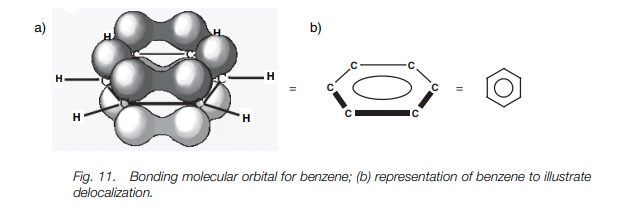Home | | Organic Chemistry | sp2 Hybridization

# sp2 HybridizationIn sp2 hybridization, a 2s orbital is ‘mixed’ with two of the 2p orbitals to form three hybridized sp2 orbitals of equal energy. A single 2p orbital is left over which has a slightly higher energy than the hybridized orbitals.

## Definition

In sp2 hybridization, a 2s orbital is ‘mixed’ with two of the 2p orbitals to form three hybridized sp2 orbitals of equal energy. A single 2p orbital is left over which has a slightly higher energy than the hybridized orbitals.

## Electronic configuration

For carbon, each sp2 hybridized orbital contains a single unpaired electron. There is also a half-filled 2p orbital. Therefore, four bonds are possible.

## Geometry

Each sp2 orbital is shaped like a deformed dumbbell with one lobe much larger than the other. The remaining 2p orbital is a symmetrical dumbbell. The major lobes of the three sp2 hybridized orbitals point to the corners of a triangle, with the 2p orbital perpendicular to the plane.

## Alkenes

Each sp2 hybridized carbon forms three σ bonds using three sp2 hybridized orbitals. The remaining 2p orbital overlaps ‘side on’ with a neighboring 2p orbital to form a pi (π) bond. The π bond is weaker than the σ bond, but is strong enough to prevent rotation of the C=C bond. Therefore, alkenes are planar, with each carbon being trigonal planar.

## Carbonyl groups

The oxygen and carbon atoms are both sp2 hybridized. The carbon has three sp2hybridized orbitals and can form threeσbonds, one of which is to theoxygen. The oxygen has one sp2 orbital which is used in the σ bond with carbon. The p orbitals on carbon and oxygen are used to form a π bond.

## Aromatic rings

Aromatic rings are made up of six sp2 hybridized carbons. Each carbon forms three σ bonds which results in a planar ring. The remaining 2p orbital on each carbon is perpendicular to the plane and can overlap with a neigh-boring 2p orbital on either side. This means that a molecular orbital is formed round the whole ring such that the six π electrons are delocalized around the ring. This results in increased stability such that aromatic rings are less reactive than alkenes.

## Aromatic rings

Conjugated systems such as conjugated alkenes and α,β-unsaturated carbonyl compounds involve alternating single and double bonds. In such systems, the p lobes of one π bond are able to overlap with the p lobes of a neighboring π bond, and thus give a small level of double bond character to the connecting bond. This partial delocalization gives increased stability to the conjugated system.

## Definition

In sp2 hybridization, the s orbital is mixed with two of the 2p orbitals (e.g. 2px and 2pz) to give three sp2 hybridized orbitals of equal energy. The remaining 2py orbital is unaffected. The energy of each hybridized orbital is greater than the original s orbital but less than the original p orbitals. The remaining 2p orbital (in this case the 2py orbital) remains at its original energy level (Fig. 1).## Electronic configuration

For carbon, there are four valence electrons to fit into the three hybridized sp2 orbitals and the remaining 2p orbital. The first three electrons are fitted into each of the hybridized orbitals according to Hund’s rule such that they are all half- filled. This leaves one electron still to place. There is  a choice between pairing it up in a half-filled sp2 orbital or placing it into the vacant 2py orbital. The usual prin- ciple is to fill up orbitals of equal energy before moving to an orbital of higher energy. However, if the energy difference between orbitals is small (as here) it is easier for the electron to fit into the higher energy 2py  orbital resulting in three half-filled sp2 orbitals and one half-filled p orbital (Fig. 1).  Four bonds are possible.

## Geometry

The 2py orbital has the usual dumbbell shape. Each of the sp2  hybridized orbitals has a deformed dumbbell shape similar to an sp3  hybridized orbital. However, the difference between the sizes of the major and minor lobes is larger for the sp2 hybridized orbital.

The hybridized orbitals and the 2py orbital occupy spaces as far apart from each other as possible. The lobes of the 2py orbital occupy the space above and below the plane of the x and z axes (Fig. 2a). The three sp2  orbitals (major lobes shown only) will then occupy the remaining space such that they are as far apart from the 2py orbital and from each other as possible. As a result, they are all placed in the xz plane pointing toward the corner of a triangle (trigonal planar shape; Fig. 2b). The angle between each of these lobes is 120 . We are now ready to look at the bonding of ansp2 hybridized carbon.## Alkenes

sp2 Hybridization results in three half-filled sp2 hybridized orbitals which form atrigonal planar shape. The use of these three orbitals in bonding explains the shape of an alkene, for example ethene (H2C=CH2). As far as the C–H bonds are concerned, the hydrogen atom uses a half-filled 1s orbital to form a strong σ bond with a half filled sp2 orbital from carbon (Fig. 3a). A strong σ bond is also possible between the two carbon atoms of ethene due to the overlap of sp2 hybridized orbitals from each carbon (Fig. 3b).The full σ bonding diagram for ethene is shown in Fig. 4a and can be simplified as shown in Fig. 4b. Ethene is a flat, rigid molecule where each carbon is trigonal pla-nar. We have seen how sp2 hybridization explains the trigonal planar carbons but we have not explained why the molecule is rigid and planar. If the σ bonds were the only bonds present in ethene, the molecule would not remain planar since rotation could occur round the C–C σ bond (Fig. 5). Therefore, there must be fur-ther bonding which ‘locks’ the alkene into this planar shape. This bond involvesthe remaining half-filled 2py orbitals on each carbon which overlap side-on to pro-duce a pi (π) bond), with one lobe above and one lobe below the plane of the mol-ecule (Fig. 6). This π bond prevents rotation round the C–C bond since the π bond would have to be broken to allow rotation. A π bond is weaker than aσ bond since the 2py orbitals overlap side-on, resulting in a weaker overlap. The presence of a π bond also explains why alkenes are more reactive than alkanes, since a π bond is more easily broken and is more likely to take part in reactions.## Carbonyl groups

The same theory explains the bonding within a carbonyl group  (C=O) where both the carbon and oxygen atoms are sp2 hybridized. The following energy level diagram (Fig. 7) shows how the valence electrons of oxygen are arranged after sp2 hybridization. Two of the sp2 hybridized orbitals are filled with lone pairs of electrons, which leaves two half-filled orbitals available for bonding. The sp2 orbital can be used to form a strong σ bond, while the 2py orbital can be used for the weaker π bond. Figure 8 shows how the σ and π bonds are formed in the carbonyl group and explains why carbonyl groups are planar with the carbon atom having a trigonal planar shape. It also explains the reactivity of carbonyl groups since the π bond is weaker than the σ bond and is more likely to be involved in reactions.## Aromatic rings

All the carbons in an aromatic ring aresp2hybridized which means that eachcarbon can form three σ bonds and one π bond. In Fig. 9a, all the single bonds are while each double bond consists of one σ bond and one π bond. However, this is an oversimplification of the aromatic ring. For example, double bonds are shorter than single bonds and if benzene had this exact structure, the ring would be deformed with longer single bonds than double bonds (Fig. 9b).In fact, the C–C bonds in benzene are all the same length. In order to understand this, we need to look more closely at the bonding which takes place. Figure 10a shows benzene with all its σ bonds and is drawn such that we are looking into the plane of the benzene ring. Since all the carbons are sp2 hybridized, there is a 2py orbital left over on each carbon which can overlap with a 2py orbital on either side of it (Fig. 10b). From this, it is clear that each 2py orbital can overlap with its neigh-bors right round the ring. This leads to a molecular orbital which involves all the 2py orbitals where the upper and lower lobes merge to give two doughnut-like lobes above and below the plane of the ring (Fig. 11a). The molecular orbital is symmetri-cal and the six π electrons are said to be delocalized around the aromatic ring since they are not localized between any two particular carbon atoms. The aromatic ring is often represented as shown in Fig. 11b to represent this delocalization of the π electrons.Delocalization increases the stability of aromatic rings such that they are less reactive than alkenes (i.e. it requires more energy to disrupt the delocalized π system of an aromatic ring than it does to break the isolated π bond of an alkene).## Conjugated systems

Aromatic rings are not the only structures where delocalization of π electrons can take place. Delocalization occurs in conjugated systems where there are alternat- ing single and double bonds (e.g. 1,3-butadiene). All four carbons in 1,3-butadiene are sp2 hybridized and so each of these carbons has a half-filled p orbital which can interact to give two π bonds (Fig. 12a). However, a certain amount of overlap is also possible between the p orbitals of the middle two carbon atoms and so the bond connecting the two alkenes has some double bond character (Fig. 12b) – borne out by the observation that this bond is shorter in length than a typical single bond. This delocalization also results in increased stability. However, it is important to realize that the conjugation in a conjugated alkene is not as great as  in the aromatic system. In the latter system, the π  electrons are completely delocalized round the ring and all the bonds are equal in length. In 1,3-butadiene, the π electrons are not fully delocalized and are more likely to be found in the ter- minal C–C bonds. Although there is a certain amount of π character in the middle bond, the latter is more like a single bond than a double bond.Other examples of conjugated systems include α,β-unsaturated ketones and α,β- unsaturated esters (Fig. 13). These too have increased stability due to conjugation.Study Material, Lecturing Notes, Assignment, Reference, Wiki description explanation, brief detail
Organic Chemistry: Structure and bonding : sp2 Hybridization |